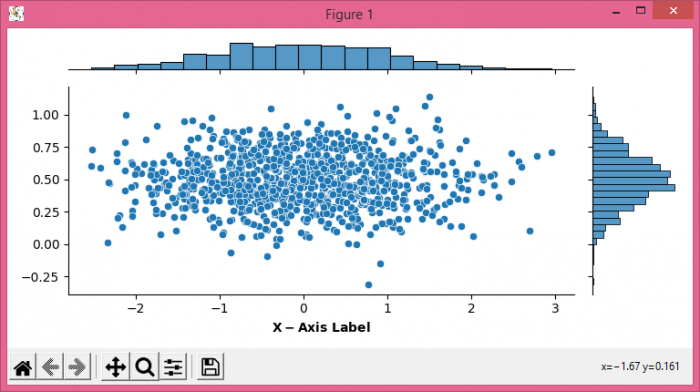# How to customize the axis label in a Seaborn jointplot using Matplotlib?

MatplotlibPythonData Visualization

To customize the axis label in a Seaborn jointplot, we can take the following steps

• Set the figure size and adjust the padding between and around the subplots.
• Create x and y data points using numpy.
• Use jointplot() method to plot a joint plot in Seaborn.
• To set the customized axis label, we can use LaTex representation or set_xlabel() method properties.
• To display the figure, use show() method.

## Example

import seaborn as sns
import numpy as np
from matplotlib import pyplot as plt
plt.rcParams["figure.figsize"] = [7.50, 3.50]
plt.rcParams["figure.autolayout"] = True
x = np.random.randn(1000,)
y = 0.2 * np.random.randn(1000) + 0.5
h = sns.jointplot(x, y, height=3.50)
h.ax_joint.set_xlabel('$\\bf{X-Axis\ Label}$')
plt.show()

## Output